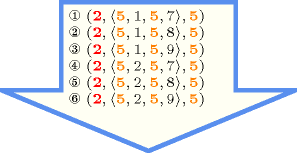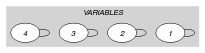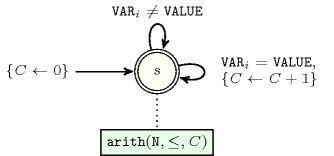## 5.36. atleast

Origin

CHIP

Constraint

$\mathrm{𝚊𝚝𝚕𝚎𝚊𝚜𝚝}\left(𝙽,\mathrm{𝚅𝙰𝚁𝙸𝙰𝙱𝙻𝙴𝚂},\mathrm{𝚅𝙰𝙻𝚄𝙴}\right)$

Synonym

$\mathrm{𝚌𝚘𝚞𝚗𝚝}$.

Arguments
 $𝙽$ $\mathrm{𝚒𝚗𝚝}$ $\mathrm{𝚅𝙰𝚁𝙸𝙰𝙱𝙻𝙴𝚂}$ $\mathrm{𝚌𝚘𝚕𝚕𝚎𝚌𝚝𝚒𝚘𝚗}\left(\mathrm{𝚟𝚊𝚛}-\mathrm{𝚍𝚟𝚊𝚛}\right)$ $\mathrm{𝚅𝙰𝙻𝚄𝙴}$ $\mathrm{𝚒𝚗𝚝}$
Restrictions
 $𝙽\ge 0$ $𝙽\le |\mathrm{𝚅𝙰𝚁𝙸𝙰𝙱𝙻𝙴𝚂}|$ $\mathrm{𝚛𝚎𝚚𝚞𝚒𝚛𝚎𝚍}$$\left(\mathrm{𝚅𝙰𝚁𝙸𝙰𝙱𝙻𝙴𝚂},\mathrm{𝚟𝚊𝚛}\right)$
Purpose

At least $𝙽$ variables of the $\mathrm{𝚅𝙰𝚁𝙸𝙰𝙱𝙻𝙴𝚂}$ collection are assigned value $\mathrm{𝚅𝙰𝙻𝚄𝙴}$.

Example
$\left(2,〈4,2,4,5〉,4\right)$

The $\mathrm{𝚊𝚝𝚕𝚎𝚊𝚜𝚝}$ constraint holds since at least 2 values of the collection $〈4,2,4,5〉$ are equal to value 4.

All solutions

Figure 5.36.1 gives all solutions to the following non ground instance of the $\mathrm{𝚊𝚝𝚕𝚎𝚊𝚜𝚝}$ constraint: ${V}_{1}\in \left[3,5\right]$, ${V}_{2}\in \left[1,2\right]$, ${V}_{3}\in \left[5,6\right]$, ${V}_{4}\in \left[7,9\right]$, $\mathrm{𝚊𝚝𝚕𝚎𝚊𝚜𝚝}$$\left(\mathbf{2},〈{V}_{1},{V}_{2},{V}_{3},{V}_{4}〉,\mathbf{5}\right)$.

##### Figure 5.36.1. All solutions corresponding to the non ground example of the $\mathrm{𝚊𝚝𝚕𝚎𝚊𝚜𝚝}$ constraint of the All solutions slotTypical
 $𝙽>0$ $𝙽<|\mathrm{𝚅𝙰𝚁𝙸𝙰𝙱𝙻𝙴𝚂}|$ $|\mathrm{𝚅𝙰𝚁𝙸𝙰𝙱𝙻𝙴𝚂}|>1$
Symmetries
• Items of $\mathrm{𝚅𝙰𝚁𝙸𝙰𝙱𝙻𝙴𝚂}$ are permutable.

• $𝙽$ can be decreased to any value $\ge 0$.

• An occurrence of a value of $\mathrm{𝚅𝙰𝚁𝙸𝙰𝙱𝙻𝙴𝚂}.\mathrm{𝚟𝚊𝚛}$ that is different from $\mathrm{𝚅𝙰𝙻𝚄𝙴}$ can be replaced by any other value.

Arg. properties

Extensible wrt. $\mathrm{𝚅𝙰𝚁𝙸𝙰𝙱𝙻𝙴𝚂}$.

Systems
Used in

implied by: $\mathrm{𝚎𝚡𝚊𝚌𝚝𝚕𝚢}$ ($\ge$ $𝙽$ replaced by $=$ $𝙽$).

Keywords
Arc input(s)

$\mathrm{𝚅𝙰𝚁𝙸𝙰𝙱𝙻𝙴𝚂}$

Arc generator
$\mathrm{𝑆𝐸𝐿𝐹}$$↦\mathrm{𝚌𝚘𝚕𝚕𝚎𝚌𝚝𝚒𝚘𝚗}\left(\mathrm{𝚟𝚊𝚛𝚒𝚊𝚋𝚕𝚎𝚜}\right)$

Arc arity
Arc constraint(s)
$\mathrm{𝚟𝚊𝚛𝚒𝚊𝚋𝚕𝚎𝚜}.\mathrm{𝚟𝚊𝚛}=\mathrm{𝚅𝙰𝙻𝚄𝙴}$
Graph property(ies)
$\mathrm{𝐍𝐀𝐑𝐂}$$\ge 𝙽$

Graph model

Since each arc constraint involves only one vertex ($\mathrm{𝚅𝙰𝙻𝚄𝙴}$ is fixed), we employ the $\mathrm{𝑆𝐸𝐿𝐹}$ arc generator in order to produce a graph with a single loop on each vertex.

Parts (A) and (B) of Figure 5.36.2 respectively show the initial and final graph associated with the Example slot. Since we use the $\mathrm{𝐍𝐀𝐑𝐂}$ graph property, the loops of the final graph are stressed in bold.

##### Figure 5.36.2. Initial and final graph of the $\mathrm{𝚊𝚝𝚕𝚎𝚊𝚜𝚝}$ constraint(a) (b)
Automaton

Figure 5.36.3 depicts the automaton associated with the $\mathrm{𝚊𝚝𝚕𝚎𝚊𝚜𝚝}$ constraint. To each variable ${\mathrm{𝚅𝙰𝚁}}_{i}$ of the collection $\mathrm{𝚅𝙰𝚁𝙸𝙰𝙱𝙻𝙴𝚂}$ corresponds a 0-1 signature variable ${S}_{i}$. The following signature constraint links ${\mathrm{𝚅𝙰𝚁}}_{i}$ and ${S}_{i}$: ${\mathrm{𝚅𝙰𝚁}}_{i}=\mathrm{𝚅𝙰𝙻𝚄𝙴}⇔{S}_{i}$. The automaton counts the number of variables of the $\mathrm{𝚅𝙰𝚁𝙸𝙰𝙱𝙻𝙴𝚂}$ collection that are assigned value $\mathrm{𝚅𝙰𝙻𝚄𝙴}$ and finally checks that this number is greater than or equal to $𝙽$.

##### Figure 5.36.3. Automaton of the $\mathrm{𝚊𝚝𝚕𝚎𝚊𝚜𝚝}$ constraint##### Figure 5.36.4. Hypergraph of the reformulation corresponding to the automaton (with one counter) of the $\mathrm{𝚊𝚝𝚕𝚎𝚊𝚜𝚝}$ constraint: since all states variables ${Q}_{0},{Q}_{1},\cdots ,{Q}_{n}$ are fixed to the unique state $s$ of the automaton, the transitions constraints share only the counter variable $C$ and the constraint network is Berge-acyclic